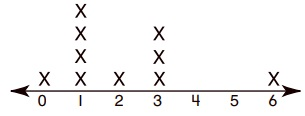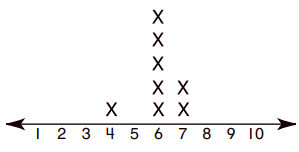# Finding the Mode and Range from a Line Plot

#### Master Mean : Learn the fundamentals of Mean Stack

59 Lectures 4 hours

#### Asp.Net Core & MERN MEAN Stack: Build Online Course and Shop

140 Lectures 38.5 hours

#### NodeJS Made Easy for MEAN or MERN Stack.

115 Lectures 6.5 hours

A line plot of a data set shows the various numbers of the data set along the x axis as per a convenient scale. It also shows the number of occurrences of each of the numbers as crosses. The smallest and largest numbers among the data set also find a place on the line plot.

By examining the line plot, we find the number with the maximum number of crosses or occurrences. This gives the mode of the data set.

We find the smallest and the largest number from the data set and find their difference and this difference is the range of the given data set.

This is how the mode and range of a data set is found from its line plot.

Find the mode and range from the following line plot### Solution

Step 1:

The number that repeats most is 1; Mode = 1

Step 2:

Range = Max number – Min number

= 6 – 0 = 6

Step 3:

Mode = 1; Range = 6

Find the mode and range from the following line plot −### Solution

Step 1:

The number that repeats most is 6; Mode = 6

Step 2:

Range = Max number – Min number

= 7 – 4 = 3

Step 3:

Mode = 6; Range = 3